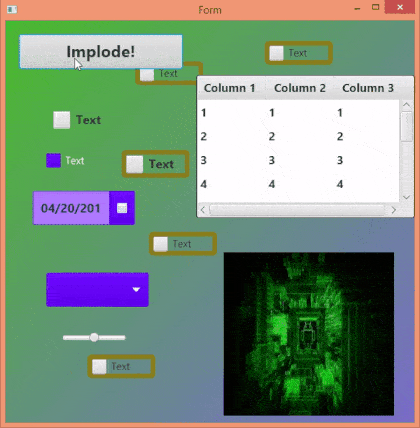# B4J Code Snippet[B4X] [BitmapCreator] Layout implode animation

The layout should be made of a "base panel" that holds all other views.
The base panel is then replaced with an ImageView and returned back at the end of the animation.
B4X:
``````'Type in globals:
Type PixelGroup (SrcX As Int, SrcY As Int, x As Float, y As Float, dx As Float, dy As Float)

Sub ImplodeLayout(BasePanel As B4XView, NumberOfSteps As Int) As ResumableSub
#if debug
Log("Animation disabled in debug mode.")
Sleep(0)
Return True
#End If
Dim scale As Float = 100dip / 100
Dim bc As BitmapCreator
Dim GroupSize As Int = 6 'you can play with this size to change the effect.
Dim iv As ImageView
iv.Initialize("")
Dim xiv As B4XView = iv
Dim bmp As B4XBitmap = BasePanel.Snapshot
BasePanel.RemoveViewFromParent

Dim w As Int = xiv.Width / scale / GroupSize
Dim h As Int = xiv.Height / scale / GroupSize
bc.Initialize(w * GroupSize, h * GroupSize)
Dim source As BitmapCreator
source.Initialize(bc.mWidth, bc.mHeight)
source.CopyPixelsFromBitmap(bmp)

Dim steps As Int = NumberOfSteps
Dim pgs(w, h) As PixelGroup
For x = 0 To w - 1
For y = 0 To h - 1
Dim pg As PixelGroup = pgs(x, y)
pg.SrcX = x * GroupSize
pg.Srcy = y * GroupSize
Select Rnd(0, 4)
Case 0
pg.x = 0
pg.y = Rnd(0, bc.mHeight)
Case 1
pg.x = bc.mWidth - 1
pg.y = Rnd(0, bc.mHeight)
Case 2
pg.x = Rnd(0, bc.mWidth)
pg.y = 0
Case 3
pg.x = Rnd(0, bc.mWidth)
pg.y = bc.mHeight - 1
End Select

pg.dx = (pg.SrcX - pg.x) / steps
pg.dy = (pg.SrcY - pg.y) / steps
Next
Next
Dim r As B4XRect
r.Initialize(0, 0, 0, 0)
For i = 0 To steps - 1
bc.FillRect(xui.Color_Transparent, bc.TargetRect)
For x = 0 To w - 1
For y = 0 To h - 1
Dim pg As PixelGroup = pgs(x, y)
pg.x = pg.x + pg.dx
pg.y = pg.y + pg.dy
r.Left = pg.SrcX
r.Right = pg.SrcX + GroupSize
r.Top = pg.SrcY
r.Bottom = pg.SrcY + GroupSize
bc.DrawBitmapCreator(source, r, pg.x, pg.y, True)
Next
Next
xiv.SetBitmap(bc.Bitmap)
Sleep(16)
Next
xiv.RemoveViewFromParent
Return True
End Sub``````

Tested in B4J. Should also work in B4A and B4i.

#### Attachments

• 1.zip
23 KB · Views: 654
Last edited:

#### Star-Dust

##### Expert
Longtime User
Can not change this?
B4X:
``Dim scale As Float = 100dip / 100``
With this?
B4X:
``Dim scale As Float = 1dip``

#### Erel

##### B4X founder
Staff member
Longtime User
No. The dip unit returns an integer (output of DipToCurrent).

•TILogistic

#### Star-Dust

##### Expert
Longtime User
Ok thanksa creative idea

Last edited:

#### Johan Hormaza

##### Well-Known Member
Longtime User
Simply magnificent, thanks @Erel#### Erel

##### B4X founder
Staff member
Longtime User
can I propose variations to this source?
Of course. You can do whatever you like with the code.

•Johan Hormaza

#### Star-Dust

##### Expert
Longtime User
these are my three variants:

Variant 1:B4X:
``````For x = 0 To w - 1
For y = 0 To h - 1
Dim pg As PixelGroup = pgs(x, y)
pg.SrcX = x * GroupSize
pg.Srcy = y * GroupSize

If (y/2)=Floor(y/2) Then
pg.x = -bc.mWidth
pg.y = pg.SrcY
Else
pg.x = bc.mWidth*2
pg.y = pg.srcy
End If

pg.dx = (pg.SrcX - pg.x) / steps
pg.dy = (pg.SrcY - pg.y) / steps
Next``````

Variant 2:B4X:
``````        For y = 0 To h - 1
Dim pg As PixelGroup = pgs(x, y)
pg.SrcX = x * GroupSize
pg.Srcy = y * GroupSize

If (y/2)=Floor(y/2) And (x/2)=Floor(x/2) Then
pg.x = -bc.mWidth
pg.y = -bc.mHeight
Else
pg.x = bc.mWidth*2
pg.y = bc.mHeight*2
End If

pg.dx = (pg.SrcX - pg.x) / steps
pg.dy = (pg.SrcY - pg.y) / steps
Next``````

Variant 3:B4X:
``````For y = 0 To h - 1
Dim pg As PixelGroup = pgs(x, y)
pg.SrcX = x * GroupSize
pg.Srcy = y * GroupSize

pg.x = pg.SrcX + Rnd(0,60) - 30
pg.y = pg.SrcY + Rnd(0,60) - 30

pg.dx = (pg.SrcX - pg.x) / steps
pg.dy = (pg.SrcY - pg.y) / steps``````

#### Johan Hormaza

##### Well-Known Member
Longtime User
these are my three variants:

Variant 1:
View attachment 66942
B4X:
``````For x = 0 To w - 1
For y = 0 To h - 1
Dim pg As PixelGroup = pgs(x, y)
pg.SrcX = x * GroupSize
pg.Srcy = y * GroupSize

If (y/2)=Floor(y/2) Then
pg.x = -bc.mWidth
pg.y = pg.SrcY
Else
pg.x = bc.mWidth*2
pg.y = pg.srcy
End If

pg.dx = (pg.SrcX - pg.x) / steps
pg.dy = (pg.SrcY - pg.y) / steps
Next``````

Variant 2:
View attachment 66943
B4X:
``````        For y = 0 To h - 1
Dim pg As PixelGroup = pgs(x, y)
pg.SrcX = x * GroupSize
pg.Srcy = y * GroupSize

If (y/2)=Floor(y/2) And (x/2)=Floor(x/2) Then
pg.x = -bc.mWidth
pg.y = -bc.mHeight
Else
pg.x = bc.mWidth*2
pg.y = bc.mHeight*2
End If

pg.dx = (pg.SrcX - pg.x) / steps
pg.dy = (pg.SrcY - pg.y) / steps
Next``````

Variant 3:
View attachment 66944
B4X:
``````For y = 0 To h - 1
Dim pg As PixelGroup = pgs(x, y)
pg.SrcX = x * GroupSize
pg.Srcy = y * GroupSize

pg.x = pg.SrcX + Rnd(0,60) - 30
pg.y = pg.SrcY + Rnd(0,60) - 30

pg.dx = (pg.SrcX - pg.x) / steps
pg.dy = (pg.SrcY - pg.y) / steps``````
Great job!!! THANK YOU!!

#### Star-Dust

##### Expert
Erel did the important job, I only modified a little bit, but the idea is his•